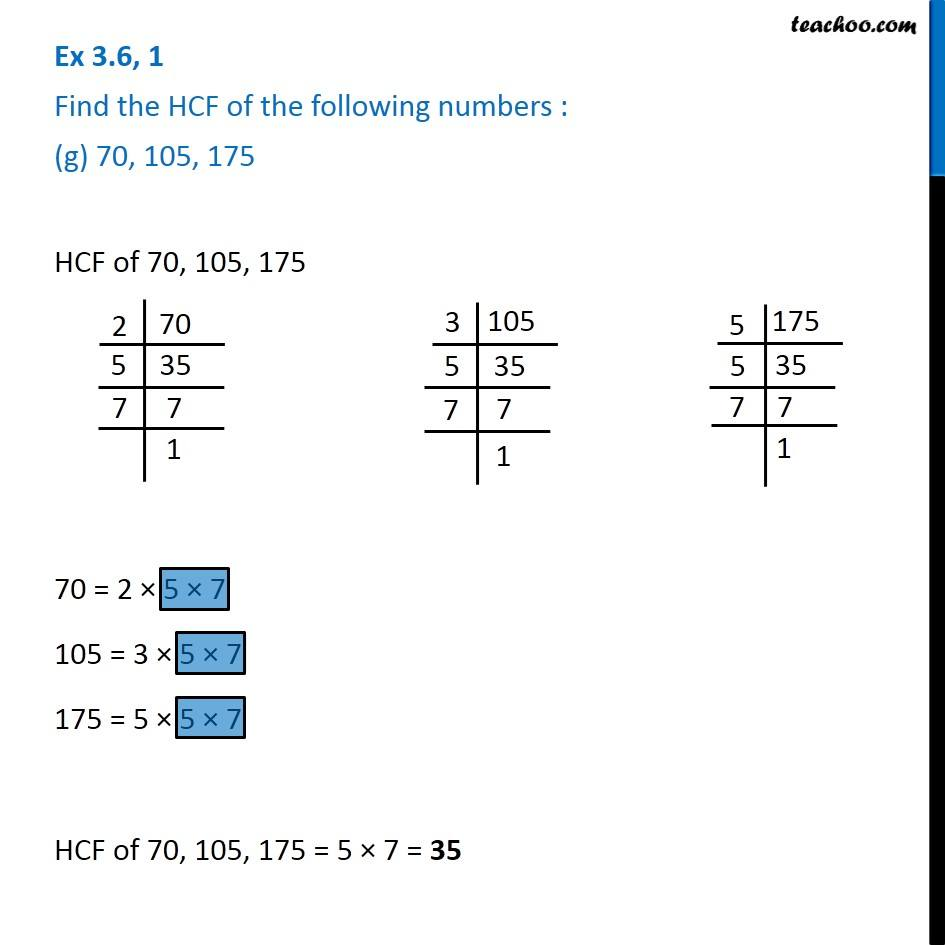1. Chapter 3 Class 6 Playing with Numbers
2. Serial order wise
3. Ex 3.6

Transcript

Ex 3.6, 1 Find the HCF of the following numbers : (g) 70, 105, 175 HCF of 70, 105, 175 70 = 2 × 5 × 7 105 = 3 × 5 × 7 175 = 5 × 5 × 7 HCF of 70, 105, 175 = 5 × 7 = 35